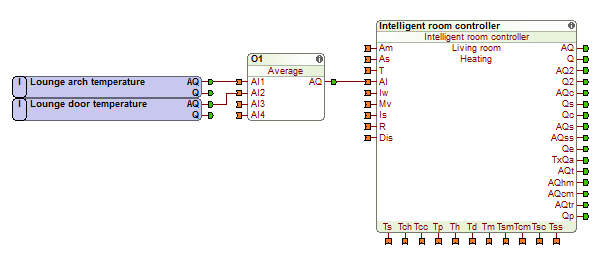# Average

## INFORMATION

The values at the four analogue AI1 to AI4 are evaluated, then added and divided by the number of used inputs. Then the mean value is output at AQ.

If an input of the function block is not connected then it is not used for the calculation.

#### EXAMPLE

In the example below the average temperature of the room is calculated. This is useful if you have a large room that you want to have two sensors in for a more accurate temperature calculation.## OVERVIEW

#### INPUTS

AI1 Analogue input 1 Connect temperature signals etc.
AI2 Analogue input 2 Connect temperature signals etc.
AI3 Analogue input 3 Connect temperature signals etc.
AI4 Analogue input 4 Connect temperature signals etc.

#### OUTPUTS

AQ Analogue output The mean of the 4 inputs is calculated and output here.